# An eccentric, compressive 1220-N force P is applied to the end of a cantilever beam. Replace P with an equivalent force-couple system at G.

Question-AnswerCategory: Engineering MechanicsAn eccentric, compressive 1220-N force P is applied to the end of a cantilever beam. Replace P with an equivalent force-couple system at G.

An eccentric, compressive 1220-N force P is applied to the end of a cantilever beam. Replace P with an equivalent force-couple system at G.Step: 1

Given that,
An eccentric compressive force at A,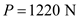Position vector of A,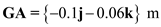Force vector at A,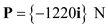Step: 2

Let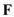andare the equivalent force and couple acting at G which are replaced by the single force at A
The equivalent force at G,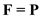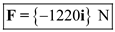Step: 3

Moment at G,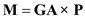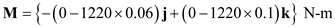The equivalent moment at G,